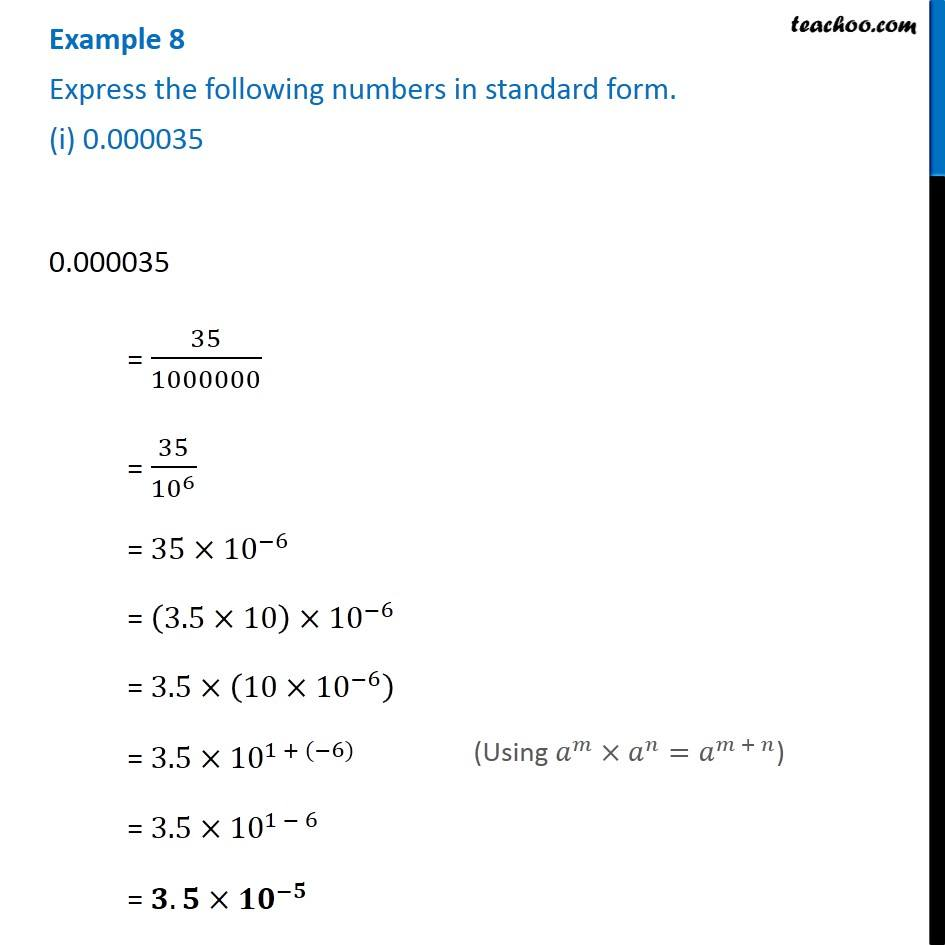Converting numbers to standard form

Chapter 10 Class 8 Exponents and Powers
Concept wiseLearn in your speed, with individual attention - Teachoo Maths 1-on-1 Class

### Transcript

Example 8 Express the following numbers in standard form. (i) 0.000035 0.000035 = 35/1000000 = 35/〖10〗^6 = 35×〖10〗^(−6) = (3.5×10)×〖10〗^(−6) = 3.5×(10×〖10〗^(−6) ) = 3.5×〖10〗^(1 + (−6)) = 3.5×〖10〗^(1 − 6) = 𝟑.𝟓×〖𝟏𝟎〗^(−𝟓) (Using 𝑎^𝑚×𝑎^𝑛=𝑎^(𝑚 + 𝑛))